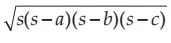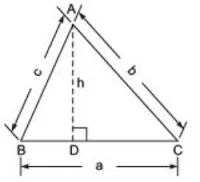## Revision Notes of Chapter 12 Heron's Formula Class 9th Math

Topics in the Chapter
• Basics Revision
• Area of Triangle
• Heron's Formula
• Area of equilateral Triangle
Basics Revision

• Area of a triangle =  1/2 × Base × Height
Heron’s formula: For sides of a triangle being a, b, c:
Area of Triangle =where s is semi-perimeter and s = (a+b+c)/2
• For finding area of a quadrilateral we divide it into various triangles. Then we use Heron’s formula to find the area of the triangles.

Area of TrianglesWe have already learnt that area of a triangle
= 1/2 × (side) × [altitude corresponding to that side (or height)]
= 1/2 × BC × AD
= 1/2 × a × h
Thus, area of a triangle = 1/2 × Base × Height

Note:
Unit of measurement for area of any plane figure is taken as square metre (m2) or square centimetre (cm2), etc.

Area of Triangle using Heron's Formula

When it is not possible to find the height of the triangle easily and measures of all the three sides are known then we use Heron’s formula, which is given by:
Area of a triangle =where a, b and c are the sides of the triangle and s = semi-perimeter, i.e. half the perimeter of the triangle = (a+b+c)/2

Area of an Equilateral Triangle

Let the side of the equilateral triangle be ‘a’.⇒ Area of the triangleThus, area of an equilateral triangle = √3/4 × side2